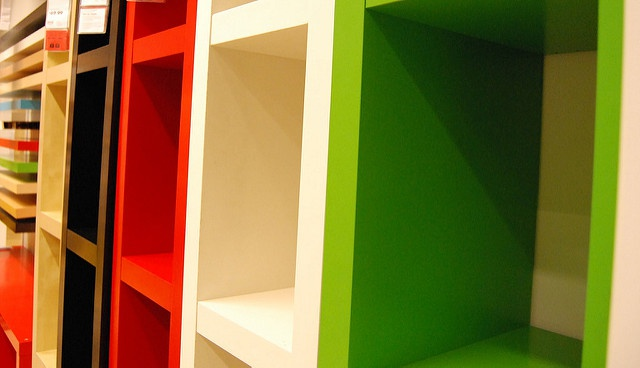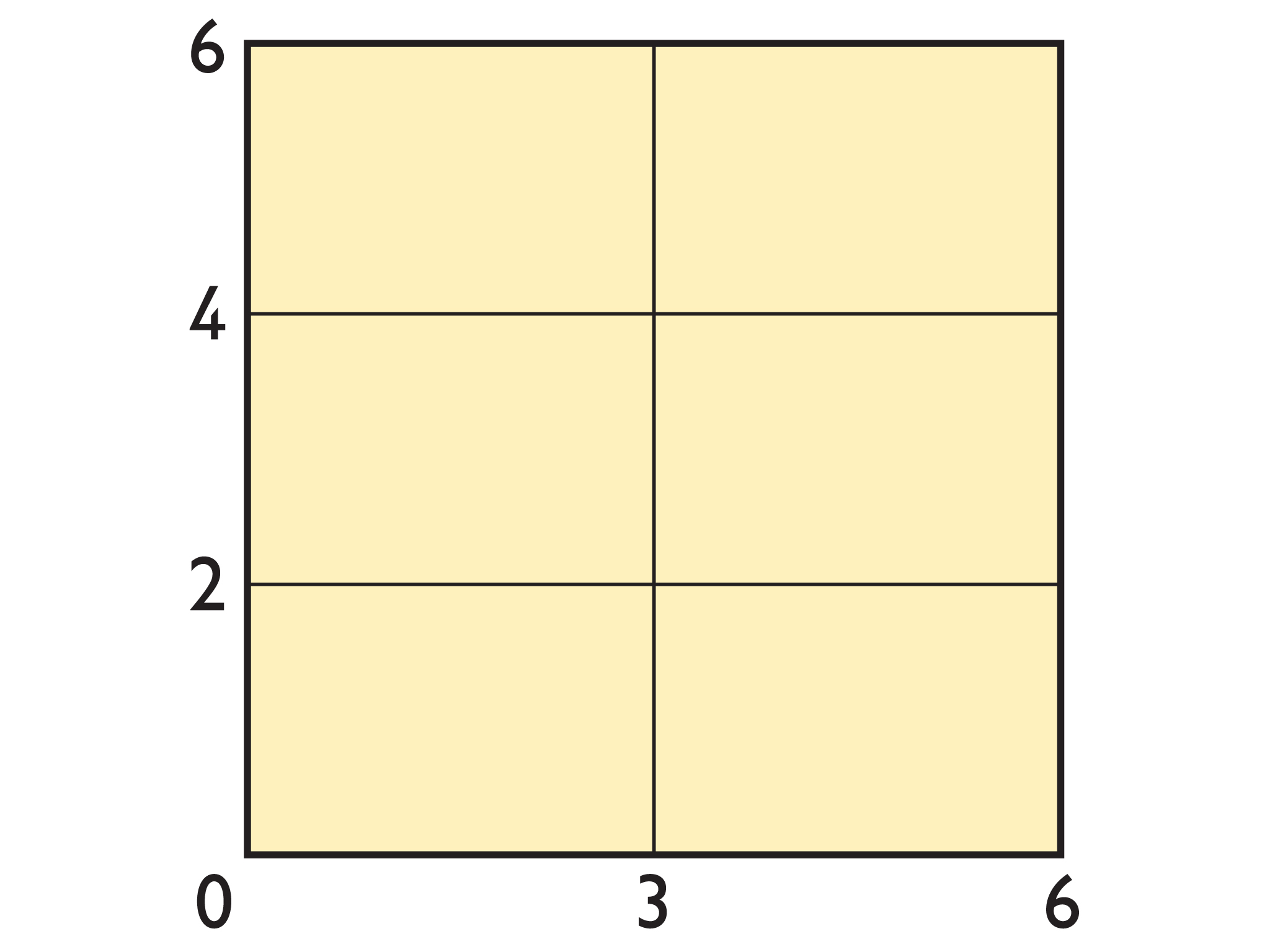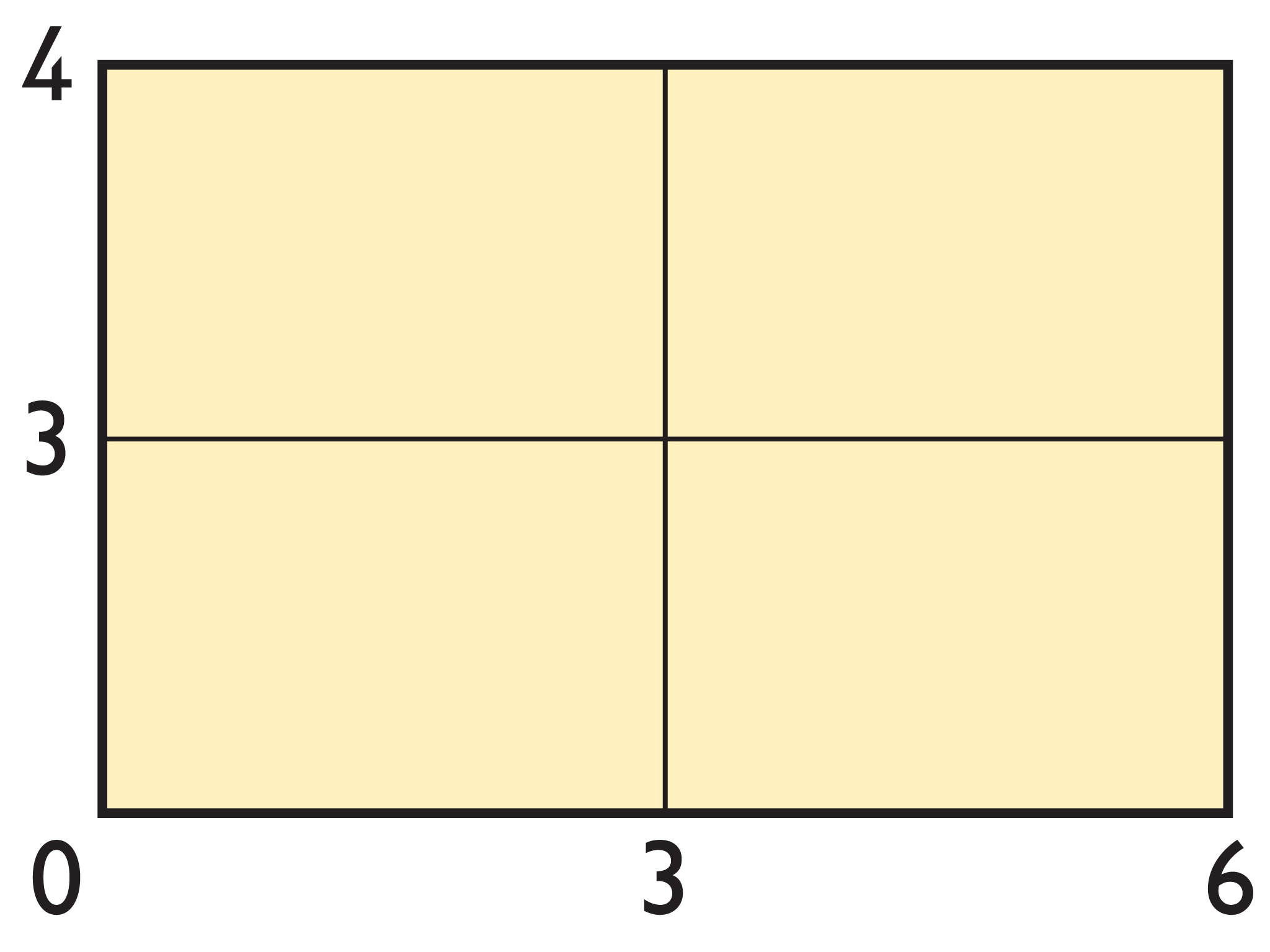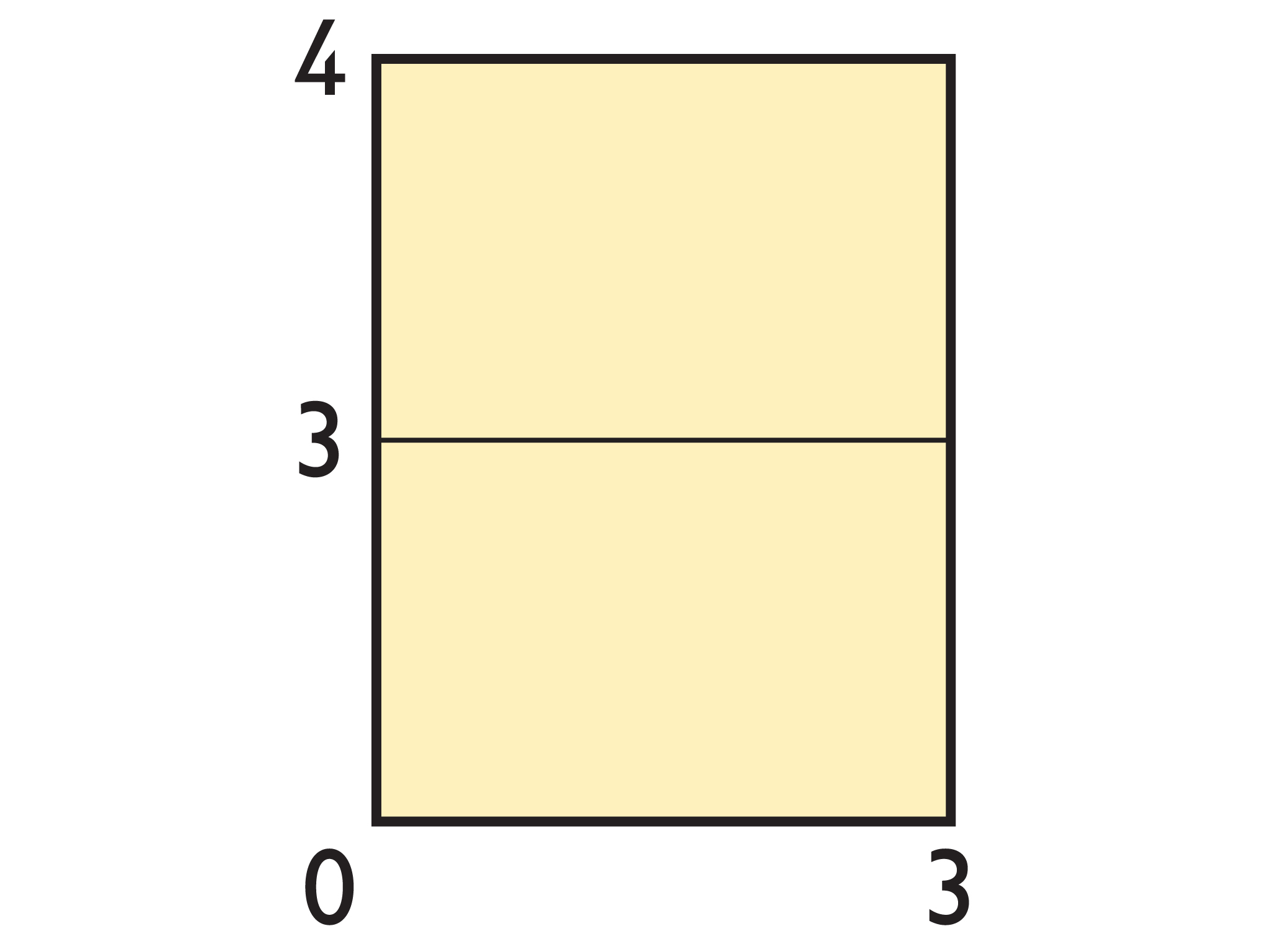Subject:
Numbers and Operations
Material Type:
Lesson Plan
Level:
Middle School
6
Provider:
Pearson
Tags:
6th Grade Mathematics, Greatest Common Factor, Models
Language:
English
Media Formats:
Interactive, Text/HTML

# Common Multiples# Lesson Overview

Students use a geometric model to investigate common multiples and the least common multiple of two numbers.

# Key Concepts

A geometric model can be used to investigate common multiples. When congruent rectangular cards with whole-number lengths are arranged to form a square, the length of the square is a common multiple of the side lengths of the cards. The least common multiple is the smallest square that can be formed with those cards.

For example, using six 4 × 6 rectangles, a 12 × 12 square can be formed. So, 12 is a common multiple of both 4 and 6. Since the 12 × 12 square is the smallest square that can be formed, 12 is the least common multiple of 4 and 6.

Common multiples are multiples that are shared by two or more numbers. The least common multiple (LCM) is the smallest multiple shared by two or more numbers.

# Goals and Learning Objectives

• Use a geometric model to understand least common multiples.
• Find the least common multiple of two whole numbers equal to or less than 12.

# Lesson Guide

Begin the class by projecting the 2 × 3 card from the interactive on the board. Show students how to make copies of the card and then give them a few minutes to make and describe squares.

The purpose of this discussion is to ensure that all students understand how to make squares with the cards. When you choose student responses to share with the class, pick responses that make this concept clear.

• Six cards can make this square:The next larger square would have side lengths of 12 units.
These errors are likely to surface:These shapes are not squares. You might suggest that students keep in mind the question, “Why squares?”

# From Rectangles to Squares

Use the 2 × 3 rectangle to do the following.

• Build at least two squares.
• Describe the squares you built.

INTERACTIVE: Build Squares with Rectangles

# Math Mission

Lesson Guide

Discuss the Math Mission. Students will find common multiples and the least common multiple of two numbers using a geometric model.

## Opening

Investigate how to find the common multiples of two numbers, and identify the least common multiple.

# Lesson Guide

Have students build squares individually.

Most likely, students will build a 12-unit square and a 24-unit square, although some students may mention squares with sides of 36 or 48 units.

• Squares will vary.

# Build Squares

Build squares using the 4 × 6 rectangle.

INTERACTIVE: Build Squares with Rectangles

## Hint:

• Start with two rectangles side by side to make a 4×12 rectangle.
• Add another row of two rectangles to make an 8×12rectangle.
• Continue adding rows of two rectangles until you make a square.

# Mathematical Practices

Mathematical Practice 4: Model with mathematics.

Students use a geometric model to identify common multiples and the least common multiple of two numbers.

Mathematical Practice 6: Attend to precision.

Watch for students who attend to precision when building their squares and when recording their results. Students who do not create squares or who do not record their results precisely will not get a correct solution.

Mathematical Practice 8: Look for and express regularity in repeated reasoning.

Identify students who find relationships between the side lengths of the rectangles and the side lengths of the squares, and apply reasoning to determine common multiples and the least common multiple of two numbers.

# Interventions

Student has an incorrect solution.

• Have you checked your work?
• How can you describe the lengths of the sides of a square? Is this shape a square? [Point to the student’s square.] Explain why or why not.
• If it is not a square, what do you need to do to make it a square?
• How can you represent the length of the square as a multiplication problem? How can you represent the width of the square as a multiplication problem?
• What do the side lengths of the squares represent?

Student has a solution.

• Describe how to use multiplication to describe the length and width of a square.
• What do the side lengths of the squares represent? Explain your thinking.
• How did you determine the smallest possible square?
• What does the smallest possible square represent?
• How could you find the least common multiple without drawing rectangles?

• The height of the smallest square made by 4 × 6 rectangles is 3 rectangles high.
• The width of the smallest square made by 4 × 6 rectangles is 2 rectangles wide.
• One side of a rectangle is shorter than the other side. The sides of a square need to be the same length, so you need more rectangles along one direction to equal the length of the rectangles along the other direction.
• The side length of the smallest square made by 4 × 6 rectangles is 12 units.
• A 12 × 12 square is the smallest square that can be made with 4 × 6 rectangles, because 12 is the first multiple of 4 that is also a multiple of 6.
• The two equal sides of the square represent the same number that both the length and the width of the rectangle must divide evenly.

# The Smallest Square

Think about the smallest square you made using the 4 x 6 rectangles.

• How many rectangles high is the square?
• How many rectangles wide is the square?
• Why did you use more rectangles in one direction than in the other direction?
• How long is one side of the square?
• How do you know that the square is the smallest square you can make?
• The side lengths of all of the squares are the common multiples of 4 and 6. The side length of the smallest square is the least common multiple (LCM) of 4 and 6. Why would you build squares rather than rectangles to find the least common multiple?

INTERACTIVE: Build Squares with Rectangles

# Preparing for Ways of Thinking

As students work, look for work that:

• Shows that the height of any square is a multiple of 4 and that the width of any square is a multiple of 6.
• States that a 12 x 12 square is the smallest square that can be made, because 12 is the first multiple of 4 that is also a multiple of 6.
• [ common error ] Makes arrangements of rectangles that have an equal number of cards going across and down (these arrangements are not squares).
• Explains the height and width with number sentences (for example, 3 x 4 = 12 and 2 x 6 = 12).
• Explains why the lesson requires building squares: We are looking for common multiples of 4 and 6. The side lengths of the squares built from 4 x 6 rectangles represent the common multiples of 4 and 6.

# Challenge Problem

• The smallest square made from 2 × 5 rectangles is a 10 × 10 square.
• Students build the 10 × 10 square to see if their prediction is correct.

# Challenge Problem

• Start with the 2 × 5 rectangle. Predict what size the smallest possible square would be that you could build using the rectangle.
• Build the square to see if your prediction is correct.

INTERACTIVE: Build Squares with Rectangles

## Hint:

What do you notice about the length of the square compared to the length of either side of the rectangle?

# Mathematics

Have students give their presentations. Focus the student discussion on noticing that for every square that students build, multiplying the edge length of the card by the number of times that card is used along one side gives the length of the square.

• How can you find the length of the side of the square?
• What do the lengths of the squares represent? Explain.
• What does the smallest square represent? Explain.
• Why is it important to build squares and not rectangles?
• What can you reason from this model?
• How would you describe what a common multiple is? What does common mean?
• How would you describe what a least common multiple is?
• How could you find the least common multiple without drawing rectangles?

Be sure students understand that the edge lengths of the squares represent the common multiples of 4 and 6, the width and length of the rectangle.

Discuss that 12 is the least common multiple (LCM) of 4 and 6, because 12 is the first multiple of 4 that is also a multiple of 6.

If you ask students how they would find the least common multiple without drawing rectangles, some students might suggest listing the first few multiples of both numbers in a table and then finding the lowest multiple that is common to both. Other students might suggest listing the multiples of one number, and then dividing each of those multiples by the other number. The first multiple that divides evenly is the least common multiple of both numbers.

ELL: Provide ELLs the opportunity to represent their thinking using diagrams if they wish to be sure that language is not getting in the way of communicating their understanding.

SWD: Write down all methods used by students for finding the least common multiple. Highlight the important parts of the discussion. Annotate the methods students used for finding the least common multiple without making squares from rectangles. Make sure all students have this information in their notebook. Some students may need copies of the notes from this portion of the lesson.

# Ways of Thinking: Make Connections

Take notes about your classmates’ thinking as to how they know the square they built is the smallest square possible.

## Hint:

• What strategy did you use to make your squares?
• How can you determine the length of your squares?
• Explain how you know that you found the smallest possible square.
• What does the length of a square represent?
• What does the length of the smallest square represent?
• Where do you see a multiple represented in your model?
• Where do you see a common multiple represented in your model?
• Where do you see the least common multiple represented in your model?

# Lesson Guide

Ask questions such as the following as students are working:

• What strategy did you use to find the least common multiple?
• Can you use a model to find the least common multiple?
• Why is this number the least common multiple of these numbers?
• How can you find the least common multiple of more than two numbers?

• The least common multiple of 4 and 6 is 12.
• The least common multiple of 18 and 36 is 36.
• The least common multiple of 20 and 36 is 180.
• The least common multiple of 12, 20, and 30 is 60.

# Least Common Multiple

Write the least common multiple of each set of numbers.

• 4, 6
• 18, 36
• 20, 36
• 12, 20, 30

## Hint:

Generate and list the multiples of each number. Look for multiples in common. Then find the smallest one.

# Mathematics

• Have pairs quietly discuss the information in the Summary.
• As student pairs discuss together, listen for students who still do not understand multiples, common multiples, and the least common multiple. Make note of any misconceptions to clarify in the class discussion.
• Discuss the Summary as a class.

SWD: Reflecting on learning can be challenging for some students. Support students who may get lost in the meaning of this task by providing specific questions to which they can respond. Some students may require sentence starters and/or paragraph frames as writing support.

Also, support students as they reflect on their learning by providing models from earlier lessons that include the elements of a complete reflection. Create a “reflection checklist” to help students to self-assess the quality of their reflections. This will help struggling writers to self-monitor their work.

# Summary of the Math: All About Multiples

• A common multiple of two or more whole numbers is a number that is a multiple of all the numbers. For example, 12 is a common multiple of 2 and 3 because 2 × 6 = 12 and 3 × 4 = 12.
• The smallest of all the common multiples of any two or more natural numbers is called the least common multiple (LCM). For example, the least common multiple of 2 and 6 is 6, because 2 × 3 = 6 and 1 × 6 = 6. (Multiples of 2 that are less than 6 are 2 and 4; those numbers are not multiples of 6. 6 is the smallest multiple of 6.)
• When you build a square using rectangles that have edges with whole-number lengths, the edge length of the square is a common multiple of the length and width of the rectangle.

## Hint:

Can you:

• Describe the relationship between the side lengths of a rectangle and the side length of a square that was built from the rectangle?
• Use the terms multiple ,common multiple , andleast common multiple ?